Home > Publications/Periodicals > The Seismological Bulletin of Japan > User's guide

# User's guide

## 1. Earthquakes

### 1.1 Numbers of earthquakes by district and magnitude

The table shown here lists numbers of precisely defined earthquakes (classified as K, k, A; see the table in Section 1.2.3) occurring in and around Japan by region and magnitude. The Unknown column shows the number of earthquakes whose magnitude could not be determined, and the total number of quakes felt (measuring 1 or more on JMA's seismic intensity scale) is displayed at the bottom. District names for epicenters are based on the specifications of Appendix 1.A.3.. Tremors with a "Near Japan" district designation occurred in regions from No. 311 to No. 341, including Sakhalin, the Kurile Islands, the Korean Peninsula, the Ogasawara Islands, Primorskii and Taiwan.

### 1.2 Earthquake parameters

#### 1.2.1 Hypocenter calculation

Hypocenters are calculated using P-wave and S-wave arrival times, and magnitudes are determined using maximum seismic wave amplitudes. Lists of seismic stations and standard seismograph response curves are shown in Appendices 1.A.1. and 1.A.2., respectively. The iterative method [Hamada et al. (1983)(2);an extension of Geiger's method [Geiger (1910)(1)]]is used to calculate hypocenter locations. The data weighting is based on the following formula, where R denotes hypocentral distance [Ueno et al. (2002)(3)]:

 For P-waves ( Wp ) Wp = Rmin 2/R 2 For S-waves ( Ws ) Ws = Wp/3 Rmin : Hypocentral distance from the station nearest the hypocenter (km) ( if Rmin ≤ 50, Rmin = 50; if Wp > 1,Wp = 1)

Data from stations displaying large travel time residuals are not used for calculation. The depth of focus is initially calculated with no restrictions. If the solution for earthquake parameters is unstable, the best solution is sought with the epicenter fixed and the depth changed in increments of 1 km. For earthquakes located in the shallow region around the Kurile Islands (see Figure 1), the focal depth is fixed at 30 km.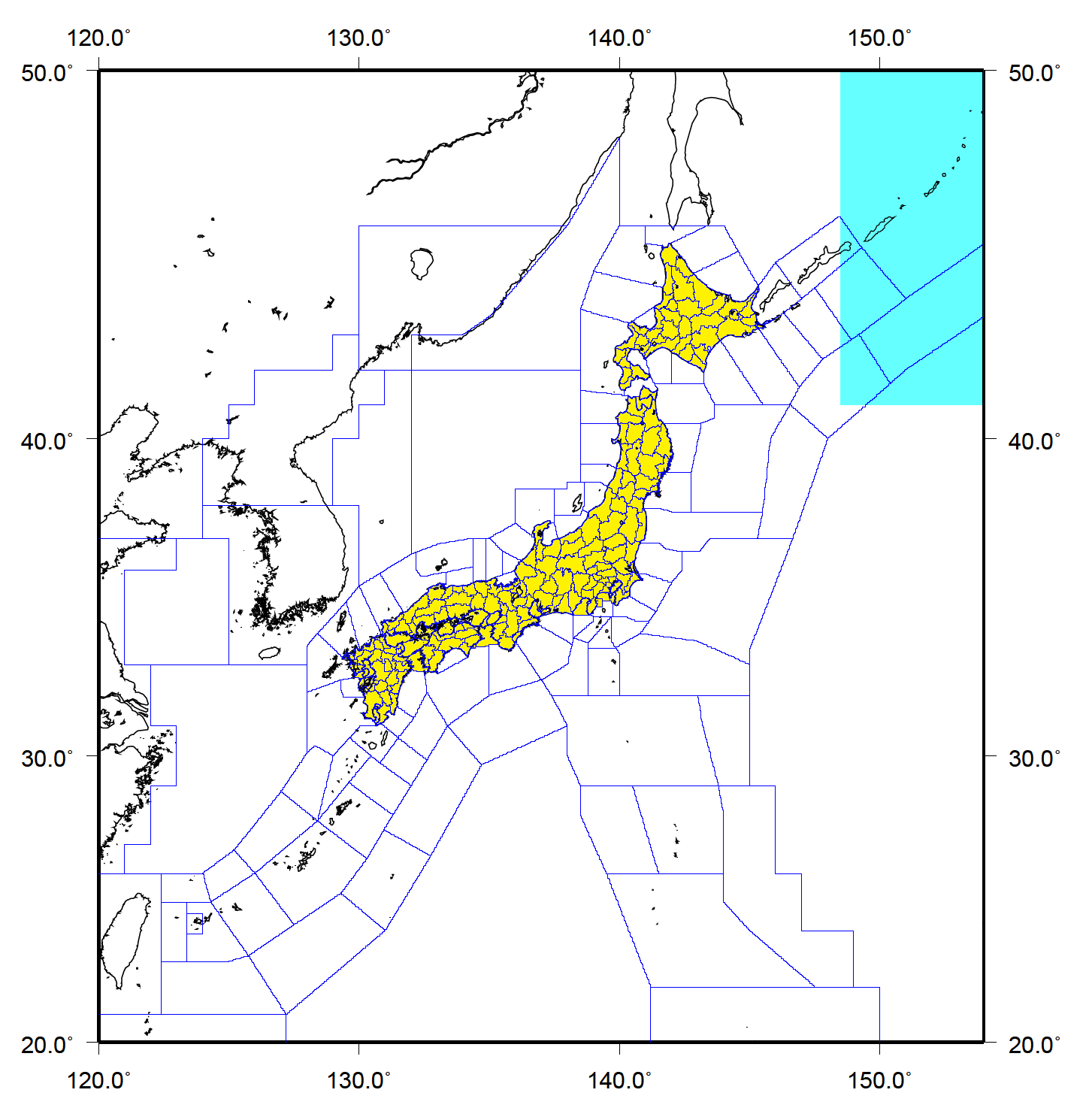Figure 1: Inland region (yellow part; depth less than 30 km) and region around the Kurile Islands (aqua-blue part; north of 41 degrees north in latitude and east of 148.5 degrees east in longitude) The blue lines show the boundaries of regions used for Earthquake Information.

The JMA2001 travel time table [Ueno et al. (2002)(3)] is mainly used for theoretical travel time calculation. For earthquakes located in the shallow region around the Kurile Islands, the travel time table given by Ichikawa (1978)(5) is used. The Jeffreys-Bullen travel time table [Jeffreys and Bullen (1958)(4)] is used for earthquakes with an epicentral distance 2,000 km or farther away from JMA's seismic network (see the travel time tables and seismic velocity structures for details).

Magnitude determination:

1. Local Meteorological Office magnitude MJ
This is calculated for large shallow (H ≤ 60km)earthquakes using acceleration data from Local Meteorological Office seismic intensity meters.
MJ is given as the average of

MJOBS=1/2log(AN2+AE 2)+1.73logΔ−0.83

using the maximum displacement amplitudes recorded at these offices [Tsuboi (1954)(7)]. Here, acceleration data are integrated twice to determine displacement, to which a high-pass (6 s) filter is applied for mechanical strong-motion seismogram simulation.
This method is provisionally used until the independence of CD in Eq.(ii) regarding the magnitude of large earthquakes (especially for M7 class or above) is confirmed.

2. Displacement Magnitude MD
This is calculated as the average of

MDST=1/2log(AN2+AE 2)+βD(Δ, H)+CD

over stations in the ranges of R ≥ 30km and Δ≤ 700km that record maximum amplitudes of horizontal displacement [Katsumata (2004)(8)]. For earthquakes located in the region of Figure 2, however, stations in the ranges of R ≥ 30km and Δ≤ 2000km are always used. If the average involves fewer than three stations, Δ is extended up to 2,000km. If only two stations are used to determine MD, the notation is Md.

3. Velocity Magnitude MV
This is calculated as the average of

MVST=α logAZ+βV (Δ, H)+CV

over stations in the ranges of R >= 5km and R <= 400km that record maximum amplitudes of velocity in the vertical component [Funasaki et al. (2004)(9)]. If the average involves fewer than four stations, Δ is extended to 1,000km. If only two or three stations are used to determine MV, the notation is Mv.

4. Moment magnitude Mw
Mw is given by the results of Centroid Moment Tensor Solutions

In the averaging procedure for ⅰ, ⅱ and ⅲ, the initial mean of magnitudes at all stations is first calculated. The mean and standard deviation of magnitudes for the stations are then calculated, with values deviating from the initial mean by more than 0.5 discarded. The mean is adopted as the magnitude only if the standard deviation is less than 0.35.

The meanings of the symbols used in the above formulas are as follows:

 H : Focal depth (km) Δ : Epicentral distance (km) R : Hypocentral distance(km) α : Constant 1/0.85=1.176 βD, βV : Terms showing dependence on Δ and H (see Figure 3 and 4) CD : Correction value (= 0.2) used for D93-type seismometers CV : Correction value depending on seismometer types (see Table 1) AN, AE : Maximum displacement amplitude in the horizontal component of D93-type seismometers. The unit is micrometers = 10−6 m. AZ : Maximum velocity amplitude in the vertical component of EMT-, EMT76- or E93-type seismometers. The unit is 10−5 m/s.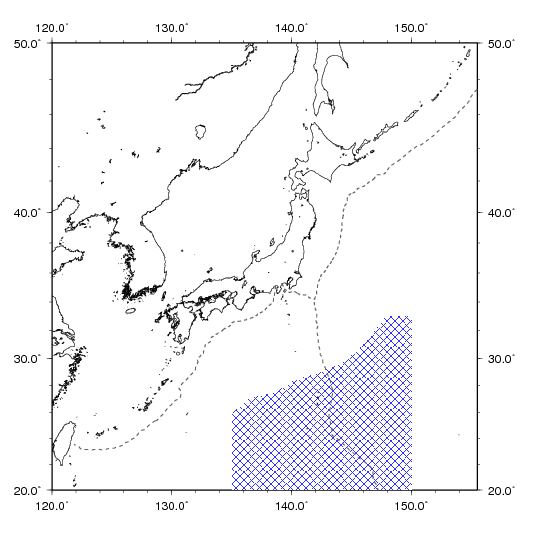Figure 2: Region(blue shading) where stations in the ranges of R ≥ 30 km and Δ≤ 2000 km are always used for Displacement Magnitude calculation.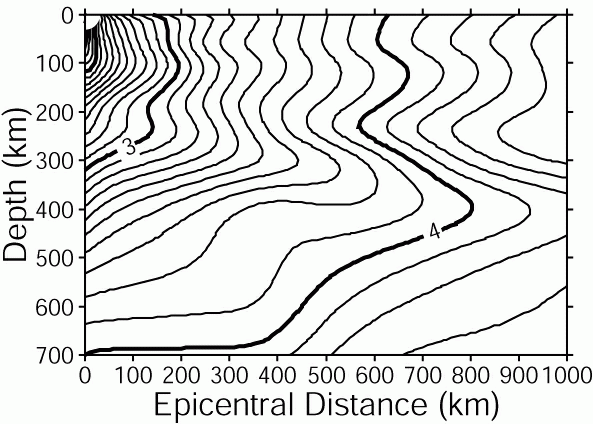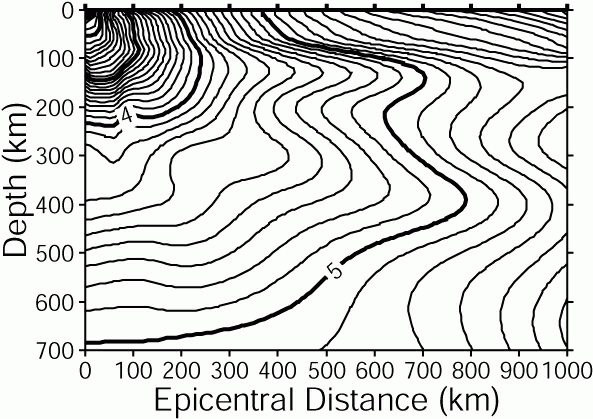Figure 3 : Contour representation of βD Figure 4 : Contour representation of βV

Seismometer type Value Seismometer type Value
JMA-93 type, velocity sensor, remote, surface installation 0.00 JMA velocity sensor, installed in volcanic region 1.13
JMA OBS, velocity sensor 0.47 Other JMA seismometer, velocity sensor −0.18
JMA-67 type, velocity sensor with logarithmic-amplifier, remote −0.02 High-sensitivity seismometer, velocity sensor, bore-hole installation 0.43
JMA velocity sensor, local, surface installation −0.02 Other organization, velocity sensor, ground installation 0.12
JMA velocity sensor, local, buried 0.50 Other organization, velocity sensor, horizontal-tunnel installation 0.30
JMA velocity sensor with logarithmic-amplifier, local, buried −0.11 Other organization, velocity sensor, bore-hole installation 0.48
JMA velocity sensor, remote, surface installation (except 93 type) 0.03 Other organization OBS, velocity sensor 0.11
JMA velocity sensor, remote, buried 0.29
Table 1: Correction values estimated for individual seismometer types (CV)

The Matched Filter (MF) method [Moriwaki (2017)(18)] was introduced in March 22 2018 to enable the detection of deep low-frequency earthquakes occurring on the plate boundary along the Nankai Trough. Automatic hypocenter determination using this approach is based on application of the four-dimensional grid search method near the detected event. If the hypocenter is determined to be at the edge of a grid space, the template event hypocenter is adopted.
Magnitude is calculated as the average of
Mv=Mtemplate+αlog(Az/Aztemplate)

Here:

 α :Constant 1/0.85=1.176 Mtemplate :Magnitudes of the template for low-frequency earthquakes Az,Aztemplate :Maximum velocity amplitude in the vertical component of detected and template low-frequency earthquakes

The notation of the magnitude determined is Mv regardless of the number of stations used for determination.

#### 1.2.2 Hypocenter determination

A new auto hypocenter detection approach (the PF method; [Tamaribuchi et al. (2016)(17)]) was adopted in April 2016, and three new hypocenter categories were introduced.

1. Fully checked hypocenter
In this category, full checking of earthquake locations and automatic phases, along with manual picking are performed by staff for earthquakes with a magnitude exceeding the threshold (Mth). This is the same as the method used until March 2016.
2. Simply checked hypocenter
In this category, simple checking of earthquake locations and automatic phases, along with some manual picking are performed by staff for earthquakes with a magnitude not exceeding the threshold (Mth).
3. Automatic hypocenter
In this category, simple checking of earthquake locations and automatic phases is performed, without manual picking by staff for earthquakes with a magnitude not exceeding the threshold (Mth).

The distribution of Mth values is shown Figure 5. The number is 1.7 on and near land, increasing to 3.5 with distance from land. For earthquakes occurring deeper than 150 km, Mth increases as shown in Table 2. For the prediction of the Tokai Earthquake, Mth for the Tokai area is smaller than conventionally set in other areas for all depths, as shown Figure 6. However, based on examination to determine the precision of results from automatic processing using the PF method, Mth values were unified on March 22, 2018.
The hypocenter determined using the MF method is always classified as C as shown above regardless of Mth values.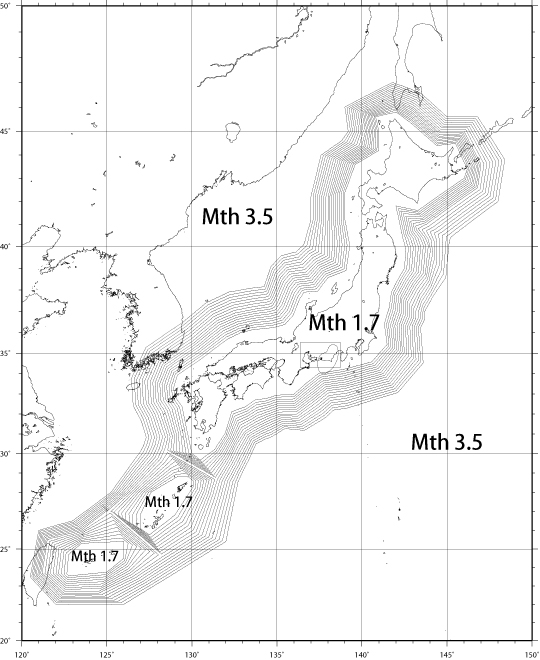Figure 5: Mth distribution (at depths from 0 to 150 km), with solid lines representing increments of 0.1 except in the Tokai district.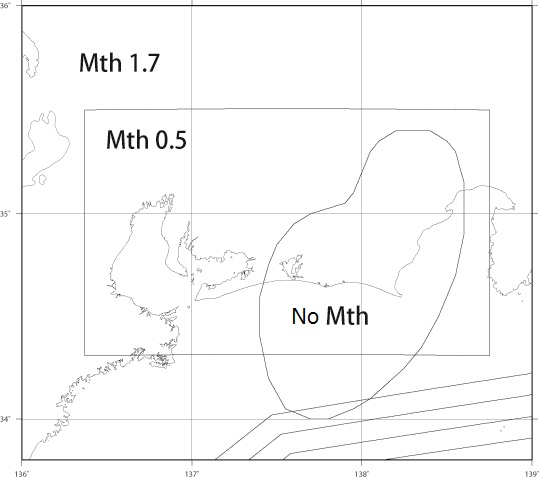Figure 6: Mth for the Tokai district (Until March 21,2018) Table 2: Mth with depth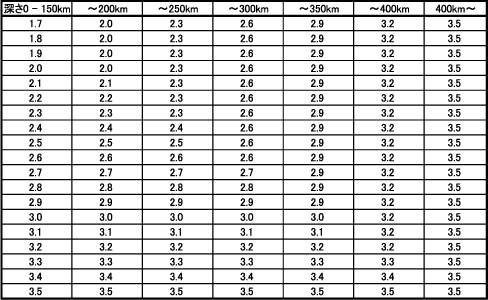If more than 40 stations report observation in fully checked hypocenter classification, the 40 nearest the focus are used for calculation (as of October 1997) [Earthquake Prediction Information Division, Seismology and Volcanology Department, Japan Meteorological Agency (1998)(6)]. Stations are selected as outlined below.

1. First, the characteristic distance (Δlim) is defined using following empirical equation:

Δlim=Δ32/100+Depth+100

Here, Δ3 denotes the epicentral distance of the third-nearest station to the epicenter, and Depth is the focal depth. The unit is kilometers.
2. Second, the 16 stations nearest the epicenter are selected. Next, 24 stations within Δlim are selected based on their epicentral distances and station quality( given as an attribute constant involving seven classes including the S/N ratio, data transmission capability and average residual travel time). If the number of selected stations are still insufficient for calculation ( in the case of an offshore or deep earthquake,for example) stations outside Δlim may be selected until an appropriate number is reached.

In simply checked hypocenter classification, all available stations are used for calculation.

In automatic hypocenter classification, the selection applied in fully checked hypocenter categorization is performed only with the PF method.

#### 1.2.3 Hypocenter classification

The calculated earthquake parameters are categorized into classes K, S, k, s, A and a based on their accuracy and determination methods.

Hypocenter classification and determination
High-precision hypocenters Low-precision hypocenters
Over Mth K (fully checked) S (fully checked)
Less than Mth k (simply cheked) A (Auto) s (simply cheked) a (Auto)

Full checking is performed for earthquakes with a seismic intensity of 1 or more in Japan, and otherwise where necessary.

The hypocenter classification standards are outlined below. The inland region is defined as shown in Figure 1, and the general region is defined as all areas except the inland region and the region around the Kurile Islands.The hypocenter determined using the MF method is classified as "a (Auto)" as shown above regardless of accuracy.

The standards for the hypocenter classification
High-precision hypocenters (K, k, A)
Region Inland General Region around the Kurile Islands
Origin time error Less than 0.5 sec Less than 1.0 sec Less than 1.5 sec
Epicenter latitude/longitude estimation error Less than 3.0 min Less than 5.0 min Less than 10.0 min

Low-precision hypocenters (S, s, a)
Region Inland General Region around the Kurile Islands
Origin time error 0.5 – 2.0 sec 1.0 – 2.0 sec 1.5 – 2.0 sec
Epicenter latitude/longitude estimation error 3.0 – 10.0 min 5.0 – 10.0 min 10.0 – 15.0 min

#### 1.2.4 Standards for posting

This bulletin lists class-K, k and A hypocenters only.

1. Origin times are given in hours, minutes and seconds, and origin time errors are given in seconds. Epicenters are given in degrees and minutes, and epicentral errors are given in minutes. Depth and depth errors are given in kilometers.
2. If the focal depth is determined with no restrictions, the focal depth error is also given. If the hypocenter is calculated with the focal depth fixed, "F" is appended to the focal depth value.
3. Magnitude is adopted as the JMA magnitude based on the priority order MJ>MD>MV>Md>Mv, and is generally noted as "MAGNITUDE1". The moment magnitude is generally noted as "MAGNITUDE2" when centroid moment tensor (CMT) solutions are determined. Magnitude types are indicated with the symbols J, D, V, d, v or W. The magnitude columns are left blank if no values are determined.
4. Maximum seismic intensity
The maximum seismic intensity on the JMA scale as observed by seismic intensity meters is denoted in column C. A, B, C and D represent five lower, five upper, six lower and six upper, respectively(See 1.A.4 for the JMA seismic intensity scale). Column C is left blank if the maximum seismic intensity is below one.
5. Epicenter region name
The district number, region number and name for the epicenter based on its latitude and longitude are given in Appendix 1.A.3 Geographical region names 1 – 8. The regions are shown in the geographical region figures (1) – (4).
6. Distant earthquakes
If seismic waves from a distant earthquake that cannot be located by JMA's network are observed, information from other bodies such as the United States Geological Survey (USGS) is carried. The notation "USGS" is placed at the end of the line in such cases. The mb (body wave magnitude) value calculated by other organizations such as USGS is generally given as the magnitude, but Ms (surface wave magnitude) or Mw (moment magnitude) values calculated by other organizations or the Mw value based on JMA's centroid moment tensor solution may be given in some cases.
7. Focal mechanism solutions
The notation "NPS" denotes that a focal mechanism solution has been determined. The solution parameters for the solution are given in 1.3 Earthquake data, and a graphical representation of solutions based on equal-area projection onto the lower hemisphere is shown in the figure 1.5 Nodal plane solutions for major earthquakes as determined from P-wave initial motion polarity and the figure 1.6 Centroid moment tensor solutions for major earthquakes.

### 1.3 Earthquake data

Earthquake* parameters and data on wave arrival at the seismic stations of JMA and other organizations** are listed.

* Earthquakes included on this list meet at least one of the following conditions:
— JMA calculates the hypocenter and magnitude
— USGS calculates the hypocenter and mb or Ms as about 6 or greater, or the tremor is felt at a Japanese station (a JMA seismic intensity of 1 or larger).

** National Research Institute for Earth Science and Disaster Prevention, Hokkaido Univ., Hirosaki Univ., Tohoku Univ., The Univ. of Tokyo, Nagoya Univ., Kyoto Univ., Kochi Univ., Kyushu Univ., Kagoshima Univ., the National Institute of Advanced Industrial Science and Technology, the Geospatial Information Authority of Japan, the Japan Agency for Marine-Earth Science and Technology, Association for the Development of Earthquake Prediction, Aomori Pref., the Tokyo Metropolitan Government, Shizuoka Pref., the Hot Springs Research Institute of Kanagawa Prefecture, Incorporated Research Institutions for Seismology

Estimated focal parameters are shown at the top of the field assigned to each event. For hypocenters with a fixed focal depth, the symbol (F) is shown after the focal depth value. Focal data with large estimation errors are reported as "POOR SOLUTION" and are excluded from 1.2 Earthquake parameters. For hypocenters determined using the MF method, the notation "MF" is placed in the first line. Template event information is also shown below the focal parameters. For distant earthquakes, parameters provided by other organizations such as USGS are included in the table. The notation "AFTER USGS" is placed in the first line in such cases. However, the Mw value based on JMA's centroid moment tensor solution may alternatively be given as the magnitude. Arrival parameters observed at each station are listed in order of epicentral distance. Arrival data on earthquakes whose focal parameters cannot be determined are given in order of the observed arrival time.

For earthquakes whose nodal plane solution or CMT solution is determined, information on the solution is shown below the focal parameters.

Any special remarks or extra information on matters such as damage and/or tsunami heights are included under "REMARK."

1. Station name (Station column)
Station codes are shown in Appendix 1.A.1 Seismic station list as JMA codes.
2. Phase name (PHA column)
Impulsive or emergent onsets of individual phases are represented by I or E at the beginning of the phase name (e.g., IP (impulsive P-wave) or ES (emergent S-wave)). The phase names APP and ASS in the PHA column represent pP and sS, respectively. The phase name M denotes data containing only the maximum displacement amplitude.
3. Residual (RES column)
The residual is the difference between observed (O) and calculated (C) travel times (O−C).
4. Maximum amplitude (N-S AMP, E-W AMP and U-D AMP columns)
1. If the maximum velocity amplitude of the vertical component is observed, its value is shown with the period of the wave portion under U-D AMP. The units for the maximum amplitude and the period are 10−5 m/s and seconds, respectively. O.S. stands for off-scale, which represents a saturated record.
The maximum velocity amplitude value and the period of the wave portion under N-S and E-W AMP are observed using an automatic processing system, and attention should be paid to these values in actual use.
2. If maximum displacement amplitudes of horizontal components from D93 seismometers are observed, the maximum amplitude value is shown with the period of the wave portion under N-S and E-W AMP and marked with an asterisk. The units for the maximum amplitude and the period are 10−6 m and seconds, respectively. The maximum amplitude is defined as half the maximum peak-to-peak amplitude on the record divided by the standard magnification.
5. Epicentral distance (Δ column)
Epicentral distance is calculated using the following formula:

cos th=sin phE sin phS+cos phE cos ph S cos(lE lS)
 where th: Epicentral angular distance (in radian) phE lE: Geocentric latitude and longitude of epicenter phS lS: Geocentric latitude and longitude of station

Δ =r th
 where Δ: Epicentral distance (km) r: Average radius of the earth (6,371.009km)
6. Azimuth (AZM column)
The azimuth from an epicenter to a station is measured clockwise from the local north.
7. Magnitude (MAG column)
Magnitudes calculated at each station are listed as station magnitudes. The calculation method is outlined in Section 1.2.3.
8. Residual of magnitude (MRES column)
The residual of magnitude is the difference between the station magnitude shown in the MAG column and the magnitude of the earthquake.

For data on events detected using the MF method, column details are as follows:

1. Station name (Station column)
2. Phase name (PHA column)
3. Head time of the time window (TIME column)
4. Correration coefficient (N-S COR, E-W COR, U-D COR column)
5. Maximum amplitude (N-S AMP, E-W AMP,U-D AMP column)
6. Epicentral distance (DELTA column)
7. Azimuth (AZM column)

### 1.4 Epicenter Distribution

Monthly and annual epicenter distributions are shown in the figures titled "Earthquake epicenters in Japan and adjacent regions" and "Regional distribution of earthquakes." Annual distributions of epicenters within certain depth layers are also shown.

### 1.5 Nodal plane solutions for major earthquakes as determined from P-wave initial motion polarity

Nodal plane solutions for major earthquakes are shown using lower-hemisphere equal-area projection. The values of their solution parameters, the strike (STR), dip (DIP) and slip-direction (SLIP) of nodal planes (NP1, NP2), and the azimuth (AZM) and plunge (PLG) of the P-, T- and N-axes are shown under each figure. The strike of each plane and the azimuth of each axis are measured clockwise from north. The dip of each plane and the plunge of each axis are measured downward from the horizontal plane. Slip signifies the motion of a hanging wall relative to a foot wall, and its direction is measured counterclockwise from the strike direction. Nodal plane solutions are determined using the method of Nakamura and Mochizuki(1998)(10), and take-off angles are calculated based on the velocity structure of Ueno et al.(2002) (3)(see the take-off angle table and seismic velocity structure).

### 1.6 Centroid moment tensor solutions for major earthquakes

CMT solutions for major earthquakes are shown using lower-hemisphere equal-area projection. The CMT solution determination method is based on Kawakatsu (1989)(11). For further details, see Nakamura et al. (2003)(12).

Information on each CMT solution is shown above and below the figure as follows:

 1st line : Origin time (JST) 2nd line : Region name Hypo. : Location (latitude, longitude, depth) of hypocenter Cent. : Location (latitude, longitude, depth) of centroid Δ t : Centroid time minus origin time (sec.) Mo : Scalar momentt Mw : Moment magnitude Mj : JMA magnitude mrr,mtt,mff,mrt,mrf,mtf : Moment tensor components STR : Strike of nodal planes (NP1, NP2) DIP : Dip angle of nodal planes (NP1, NP2) SLIP : Slip angle of nodal planes (NP1, NP2) MOM : Moment tensor component of P-, T- and N-axes AZM : Azimuth of P-, T- and N-axes PLG : Plunge of P-, T- and N-axes V.R. : Variance reduction ε : Non-double couple component ratio N : Number of stations used COMP : Number of components used

The moment tensor is expressed by the spherical polar coordinates (r, t, f), which represent upward, southward and eastward positive respectively.

The strike of each nodal plane and the azimuth of each axis are measured clockwise from north. The dip of each nodal plane and the plunge of each axis are measured downward from the horizontal plane. Slip signifies the motion of a hanging wall relative to a foot wall, and its direction is measured counterclockwise from the strike direction.

## 2. Crustal strain

Dilatational strain is continuously observed using borehole strain meters [Seismological Division, Observation Department (1979)(13), Nihei et al. (1987)(14)] and multi-component strainmeters [Ishii et al. (1992)(15)] in the Tokai and southern Kanto regions. The "Volume strain observation stations" and "Linear strain observation stations" tables in Appendix 2.A give station names, station codes, latitudes, longitudes, altitudes and depths of strain meters. The "Directions of measurement" table in Appendix 2.A gives sensor names and directions of measurement. The distribution of stations is shown in the figure "Crustal strain station map".

### 2.1 Hourly cumulative data from borehole volume strainmeters

The data consist of hourly means of strain values. Positive and negative values correspond to crustal expansion and contraction, respectively, relative to the value at the time when observation was started.
An asterisk indicates that the value has been modified from the original. Some values are accompanied by one of the following:

 LOSS Loss of data V.O. Valve Open I Instrumental adjustment P Power failure O Others

### 2.2 Volume strain change

1. Hourly data on crustal strain
Changes in hourly mean crustal strain data are shown. ATM means hourly atmospheric pressure, and PREC means precipitation per hour.
2. Secular crustal strain variations and annual crustal strain variations
Daily mean strain data for the last seven years and for one year are shown. ATM means daily mean atmospheric pressure, and PREC means daily precipitation.
3. The effects of atmospheric pressure change are removed from the data [Hikawa et al. (1983)(16)].
4. Upward and downward directions represent crustal expansion and contraction, respectively.

### 2.3 Hourly cumulative strain data from multi-component strainmeters

The data consist of hourly means of linear strain for each component. Positive and negative values correspond to crustal expansion and contraction, respectively, relative to the value at the time when observation was started.
An asterisk indicates that the value has been modified from the original. Some values are accompanied by one of the following:

 LOSS Loss of data Z.S. Zero Shift I Instrumental adjustment P Power failure O Others

### 2.4 Linear strain change

1. Hourly data on crustal strain
Changes in hourly mean crustal strain data are shown. ATM means hourly atmospheric pressure, and PREC means precipitation per hour.
2. Annual crustal strain variations
Daily mean strain data for the last year are shown. ATM means daily mean atmospheric pressure, and PREC means daily precipitation.
3. Upward and downward directions represent crustal expansion and contraction, respectively.

## References

1. Geiger(1910): Nachr.Koeninglichen Ges. Wiss. Göttingen, 4, 331pp.
2. Hamada, N., A. Yoshida and H. Hashimoto (1983): Improvement of the Hypocenter Determination Program of the Japan Meteorological Agency (Reanalyses of the Hypocenter Distribution of the 1980 Earthquake Swarm off the east coast of the Izu Peninsula and the Matsushiro Earthquake Swarm) (in Japanese), Quart. J. Seis., 48, 35 – 55.
3. Ueno, H., S. Hatakeyama, T. Aketagawa, J. Funasaki and N. Hamada (2002): Improvement of hypocenter determination procedures in the Japan Meteorological Agency (in Japanese), Quart. J. Seis., 65, 123 – 134.
4. Jeffreys S. H. and K. E. Bullen(1958): Seismological Tables, Brit. Assn. Advans., Gray-Milne Trust.
5. Ichikawa, M.(1978): A new subroutine program for determination of earthquake parameters and local travel time tables for events near the southern Kurile trench (in Japanese), Quart. J. Seis., 43, 11 – 19.
6. Earthquake Prediction Information Division, Seismology and Volcanology Department, Japan Meteorological Agency (1998): Manual for analysis, Seismological and volcanological technical report (in Japanese),72, 37 – 82.
7. Tsuboi, C.(1954): Determination of the Gutenberg-Richiter's magnitude of shallow earthquakes occurring in and near Japan (in Japanese), Zisin2, 7, 185 – 193.
8. Katsumata, A.(2004): Revision of the JMA Displacement Magnitude(in Japanese), Quart. J. Seis., 67, 1 – 10.
9. Funasaki, J. and Earthquake Prediction Information Division (2004): Revision of the JMA Velocity Magnitude(in Japanese), Quart. J. Seis., 67, 11 – 20.
10. Nakamura, M. and E. Mochizuki (1988): Focal mechanism solutions and their reliability determined by P-wave first motions (in Japanese),  Quart. J. Seis., 52, 1 –14.
11. Kawakatsu, H. (1989): Centroid single force inversion of seismic waves generated by landslides, J.Geophys.Res., 94, 12363 – 12374.
12. Nakamura, K., S. Aoki, and Y. Yoshida (2003): Centroid Moment Tensor Analysis by using the JMA broadband Seismic Observation Network (in Japanese), Quart. J. Seis., 66, 1 – 15.
13. Seismological Division, Observation Department (1979): Continuous observation of crustal movement and borehole strainmeters (1), (2) (in Japanese), Weather Service Bulletin (Sokko-Jiho), 46, 9 – 25, 107 – 111.
14. Nihei, S., O. Kamigaichi, K. Sato (1987): On the observation of volume strainmeter, based on the data of 1976 to 1986 (1) (in Japanese), Quart. J. Seis., 50, 65 – 88.
15. Ishii, H., S. Matsumoto, Y. Hirata, T. Yamauchi, T. Takahashi, K. Suzuki, S. Watanabe, T. Wakasugi, T. Kato, S. Nagao(1992): Development of New Multi-Component Small Borehole Strainmeter and Observation (in Japanese), Japan Earth and Planetary Science Joint Meeting, 1992,C22 – 03.
16. Hikawa H., K. Sato, S. Nihei, A. Fukutome, H. Takeuchi and I. Furuya (1983): Correction due to atmospheric pressure changes of data of borehole volume strainmeter (in Japanese), Quart. J. Seis., 47, 91 – 111.
17. Tamaribuchi K., K. Moriwaki, H. Ueno and S. Tsukada (2016): Automatic hypocenter determination for the seismological bulletin of Japan using Bayesian estimation (in Japanese), Quart. J. Seis., 79, 1 – 13.
18. Moriwaki K (2017): Automatic Detection of Low-Frequency Earthquakes in Southwest Japan Using Matched-Filter Technique (in Japanese), Quart. J. Seis., 81:3.